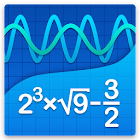Graphing Calculator + Math, Algebra & Calculus

All Android applications categories

All Android games categories# Graphing Calculator + Math, Algebra & Calculus

78K 8.3

8.3 Expert
rating

## Graphing Calculator + Math, Algebra & Calculus's review

Published: 2013-10-08, by Peter Warrior.

## A comprehensive scientific calculator app

8.3
• It does whatever you may need
• Smooth UI
• No complaints

## "Rocket scientists wanted"

Yes, your droid comes with a calculator and you might even already have an additional calculator app. However, if you are surviving high school or are in any scientific degree in college, those toy calculators aren't enough. You need to solve quadratic equations and polynomials and vectors and matrixes and draw graphs and all that odd Math stuff we mere mortals can't understand. Perhaps you need to save it all and bring it with you to your PC.

Graphing Calculator is an insanely complete app, thought for helping any need any algebra student may need. It also comes with a smooth interface and a supporting website and email address.

This free version is ad-supported and can't be run offline.

Tags: mathlab graphing calculator pro apk , matlab graphing calculator , graphing calculator by mathlab , graphing calculator by matlab apk , mathlab calc , matlab calculator apk , x2,cosx,sqrt25x^2,sqrt1002x^2 , mathlab calculator , matlab calculatorby Peter , Appszoom

Oct 08, 2013

Description

Graphing Calculator by Mathlab is a scientific graphing calculator integrated with algebra and is an indispensable mathematical tool for students from high school to those in college or graduate school, or just anyone who needs more than what a basic calculator offers. It is designed to replace bulky and costly handheld graphing calculators and works on virtually any Android phone or tablet.

Video: https://youtu.be/6BR8Lv1U9kA
Help site with instructions and examples: http://help.mathlab.us
If you have a question, send email to calc@mathlab.us

SCIENTIFIC CALCULATOR
• Square root, cube and higher roots (hold √ key)
• Exponent or power, use x^ key, (x^2)
• Logarithms ln(), log(), log[base]()
• Trigonometric functions sin π/2, cos 30°, ...
• Hyperbolic functions sinh, cosh, tanh, ... (hold "e" key to switch)
• Inverse functions (hold direct function key)
• Complex numbers, all functions support complex arguments
• Derivatives sin x' = cos x, ... (hold x^ key)
• Scientific and engineering notation (enable in menu)
• Percent mode
• Binary, octal and hexadecimal numbers, 0b1010, 0o123, 0xABC

GRAPHING CALCULATOR
• Multiple functions graphing
• Implicit functions up to 2nd degree (ellipse 2x^2+3y^2=1, etc.)
• Polar graphs (r=cos2θ)
• Parametric functions, enter each on new line (x=cos t, y=sin t)
• Function roots and critical points.
• Graph intersections
• Tracing function values and slopes
• Slide to scroll
• Pinch to zoom
• Fullscreen graphs (PRO)
• Function tables
• Save graphs as images
• Save tables as csv
• 3D graphs (PRO)

FRACTION CALCULATOR
• Simple and complex fractions 1/2 + 1/3 = 5/6
• Mixed numbers, use space to enter values 3 1/2
• Use parentheses (1+2)/(3+4)=3/7

ALGEBRA CALCULATOR
• Linear equations x+1=2 -> x=1
• Quadratic equations x^2-1=0 -> x=-1,1
• Approximate roots of higher polynomials
• Systems of linear equations, write one equation per line, x1+x2=1, x1-x2=2
• Polynomial long division
• Polynomial expansion, factoring

PROBABILITY AND STATISTICS
• Hold n! key to enter functions, double tap dot to enter comma
• Combinations nCr(5,2)=10
• Permutations nPr(5,2)=20
• Sample A=[1 2 3 4 5]
• Sample B=[2 3 4 5 6]
• Sum sum(A) = 15
• Average avg(A) = 3
• Median median(A) = 3
• Sample variance var(A) = 2.5
• Population variance varp(A) = 2
• Standard deviation stdev(A) = 1.58..., stdevp(A) = 1.41...
• Covariance cov(A,B) = 2.5
• Correlation corr(A,B) = 1

MATRIX CALCULATOR
• Matrix and vector arithmetic operations
• Vector cross product, dot product (hold *) and norm
• Matrix determinant, inverse, transpose and trace functions

LIBRARY
• Physical constants (PRO)
• User defined constants and functions (PRO)
• Save expressions for future reference

## Users review

from 78.019 reviews

"Awesome"

9